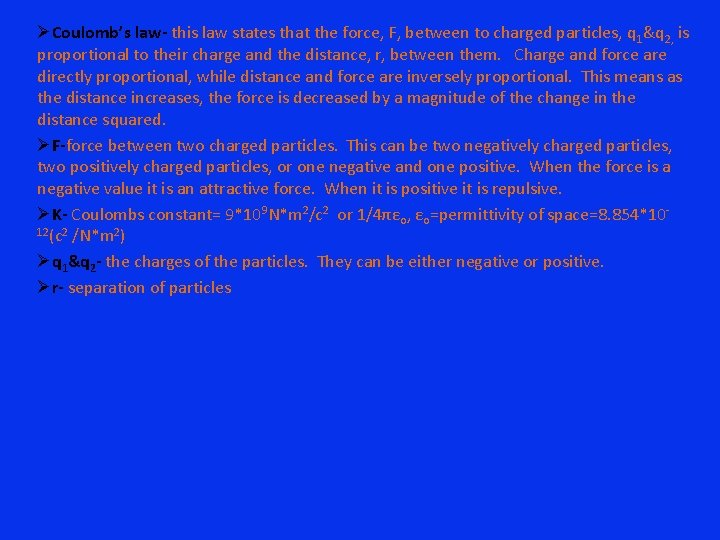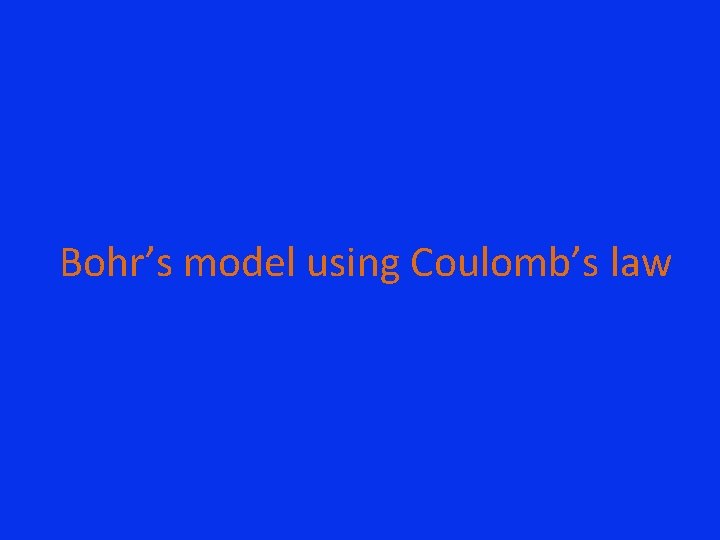# Coulombs law Coulombs law this law states that

• Slides: 6Coulomb’s lawØCoulomb’s law- this law states that the force, F, between to charged particles, q 1&q 2, is proportional to their charge and the distance, r, between them. Charge and force are directly proportional, while distance and force are inversely proportional. This means as the distance increases, the force is decreased by a magnitude of the change in the distance squared. ØF-force between two charged particles. This can be two negatively charged particles, two positively charged particles, or one negative and one positive. When the force is a negative value it is an attractive force. When it is positive it is repulsive. ØK- Coulombs constant= 9*109 N*m 2/c 2 or 1/4πεo, εo=permittivity of space=8. 854*1012(c 2 /N*m 2) Øq 1&q 2 - the charges of the particles. They can be either negative or positive. Ør- separation of particlesBohr’s model using Coulomb’s lawBohr’s model continued ØRutherford’s theory of electron’s revolving around the nucleus, in various orbits, explains why we see a line spectra for hydrogen. However this theory did not account for why the electron does not collapse into the nucleus. According to a classical theory, “an accelerated particle radiates energy (as electromagnetic waves). Thus, an electron in a Rutherford orbit, circulating at constant speed but with a continually changing direction of its velocity vector is being accelerated; thus, the electron should constantly lose energy by radiating and spiral into the nucleus. ”(hydro re visit)Bohr’s continued ØBohr, however, found a way to explain line spectra and why the electron was not spiraling into the nucleus. He theorized quantized energy levels, and the electrons ability to only move between these energy states. Bohr’s model states that the electron is held into orbit by coulombic forces of attraction, between the electron and proton of the positively charged nucleus, and the centrifugal force of the electron. ØThis results in the equations ke 2/r 2 and mev 2/r equal to each other, k. Ze 2/r 2= mev 2/r ØCoulomb’s law, kq 1 q 2/r 2, is rewritten as ke 2/r 2. Where “e” is the charge of the particle, k is Coulomb’s constant, Z is atomic number, and r is the distance the electron is from the nucleus. ØFor the centrifugal force equation, mev 2/r , me is the mass of the electron, v is the velocity of the electron, and r is the radius of the electron’s orbit, around the nucleus.A one electron atom ØIn a one electron atom q 1&q 2 are replaced with e 2 and r is now the distance between orbiting electron and the nucleus, also known as the radius. ØIn terms of the equation k. Ze 2/r 2 q 1&q 2 are replaced with e and Z. Z represents the atomic number, or the number of protons in the nucleus. This is also the positive charge that pulls the electron, e, toward the nucleus. r represents the radius of the electrons orbit, or the distance between the electron and the proton in the nucleus.